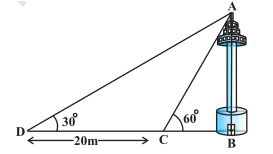# 11. A TV tower stands vertically on a bank of a canal. From a point on the other bank directly opposite the tower, the angle of elevation of the top of the tower is 60°. From another point 20 m away from this point on the line joing this point to the foot of the tower, the angle of elevation of the top of the tower is 30° (see Fig. 9.12). Find the height of the tower and the width of the canal.M manishSuppose the  is the height of the tower AB and BC =  m
It is given that, width of CD is 20 m,
According to question,

In triangle ,
............(i)

In triangle ACB,
.............(ii)

On equating eq (i) and (ii) we get:

from here we can calculate the value of    and the width of the canal is 10 m.

Exams
Articles
Questions